Chapter 3 Stoichiometry Calculations with Chemical Formulas and

• Slides: 71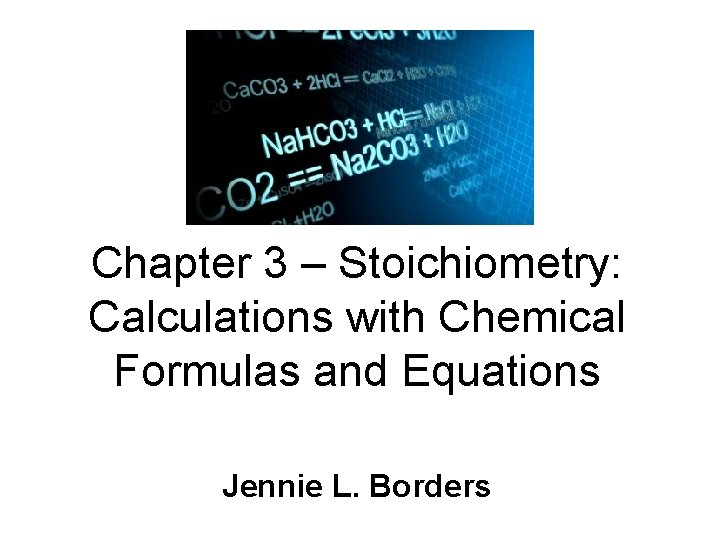Chapter 3 – Stoichiometry: Calculations with Chemical Formulas and Equations Jennie L. Borders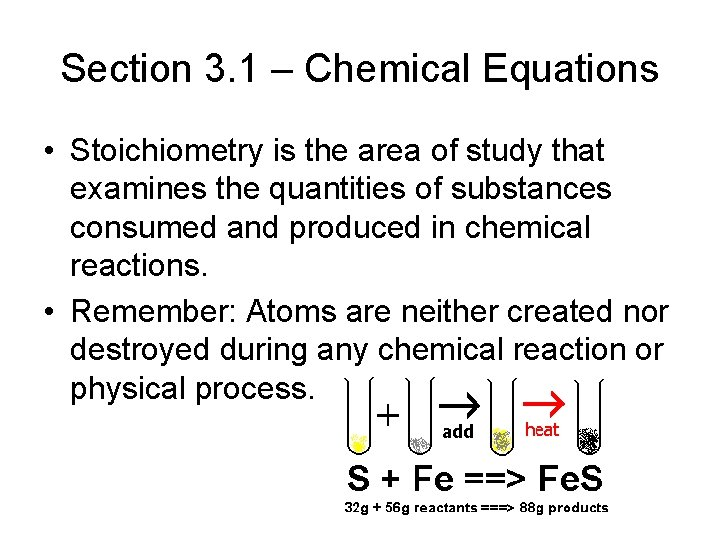Section 3. 1 – Chemical Equations • Stoichiometry is the area of study that examines the quantities of substances consumed and produced in chemical reactions. • Remember: Atoms are neither created nor destroyed during any chemical reaction or physical process.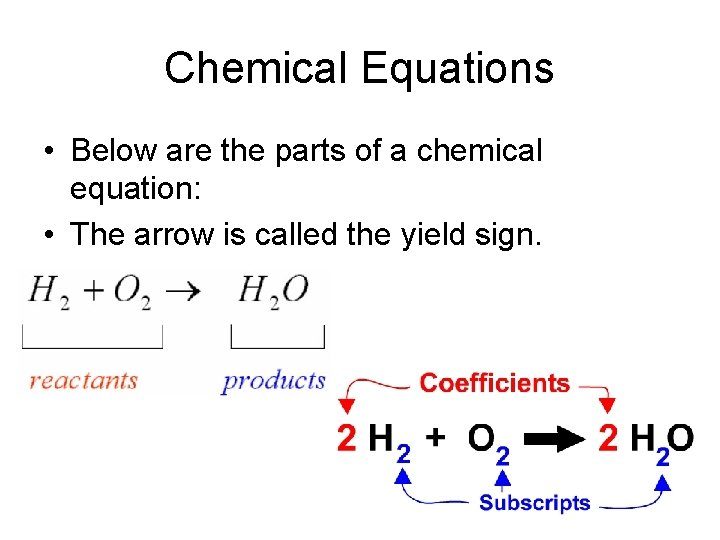Chemical Equations • Below are the parts of a chemical equation: • The arrow is called the yield sign.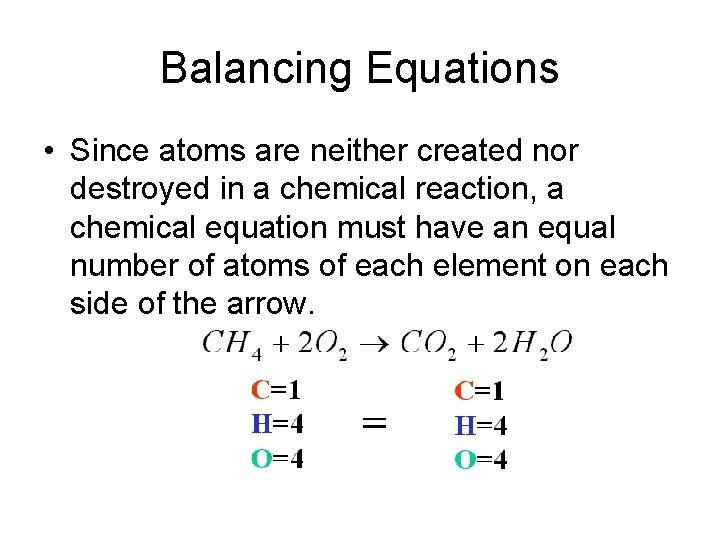Balancing Equations • Since atoms are neither created nor destroyed in a chemical reaction, a chemical equation must have an equal number of atoms of each element on each side of the arrow.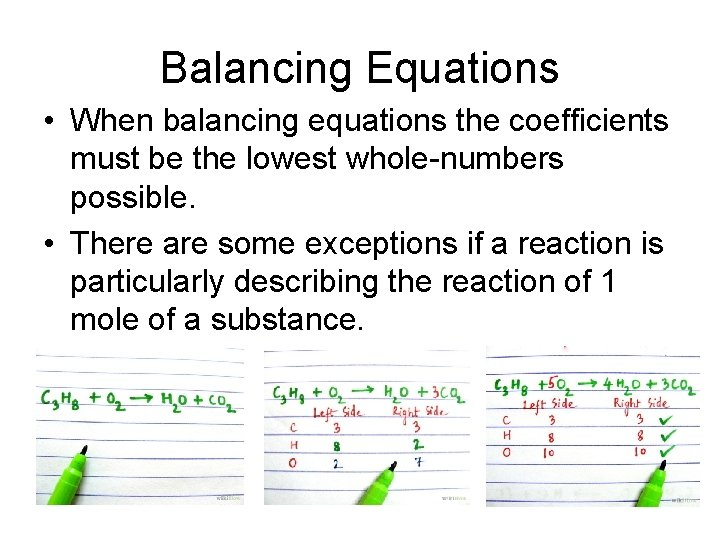Balancing Equations • When balancing equations the coefficients must be the lowest whole-numbers possible. • There are some exceptions if a reaction is particularly describing the reaction of 1 mole of a substance.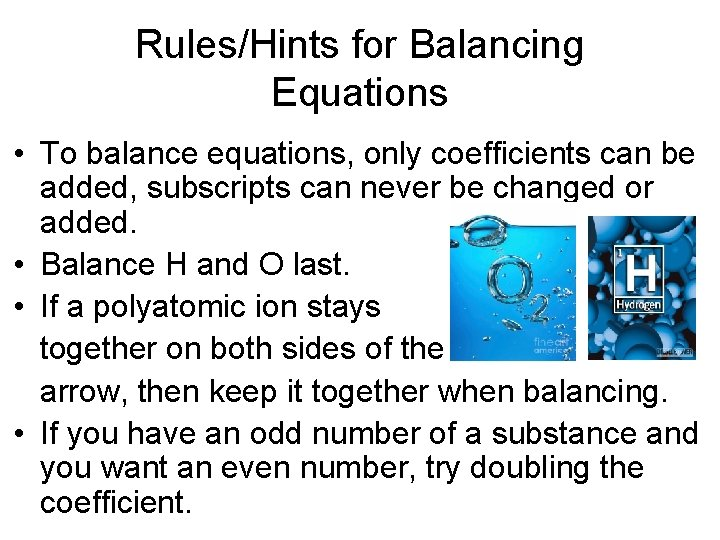Rules/Hints for Balancing Equations • To balance equations, only coefficients can be added, subscripts can never be changed or added. • Balance H and O last. • If a polyatomic ion stays together on both sides of the arrow, then keep it together when balancing. • If you have an odd number of a substance and you want an even number, try doubling the coefficient.Sample Exercise 3. 1 • The following diagram represents a chemical reaction in which the red spheres are oxygen atoms and the blue spheres are nitrogen atoms. a. Write the chemical formulas for the reactants and products.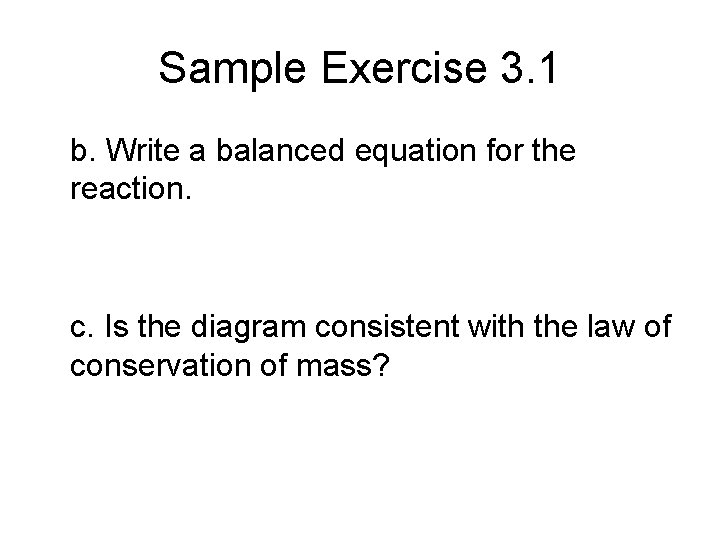Sample Exercise 3. 1 b. Write a balanced equation for the reaction. c. Is the diagram consistent with the law of conservation of mass?Practice Exercise • In the following diagram, the white spheres represent hydrogen atoms, and the blue sphere represent nitrogen atoms. To be consistent with the law of conservation of mass, how many NH 3 molecules should be shown in the right box?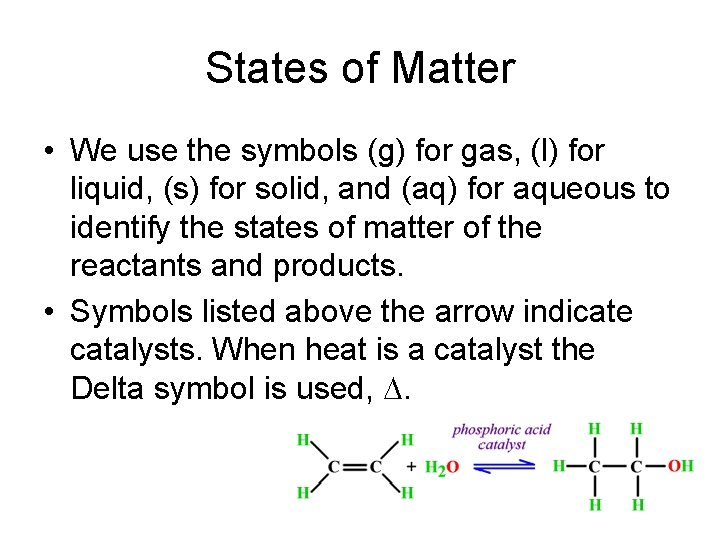States of Matter • We use the symbols (g) for gas, (l) for liquid, (s) for solid, and (aq) for aqueous to identify the states of matter of the reactants and products. • Symbols listed above the arrow indicate catalysts. When heat is a catalyst the Delta symbol is used, D.Sample Exercise 3. 2 • Balance this equation: Na(s) + H 2 O(l) Na. OH(aq) + H 2(g)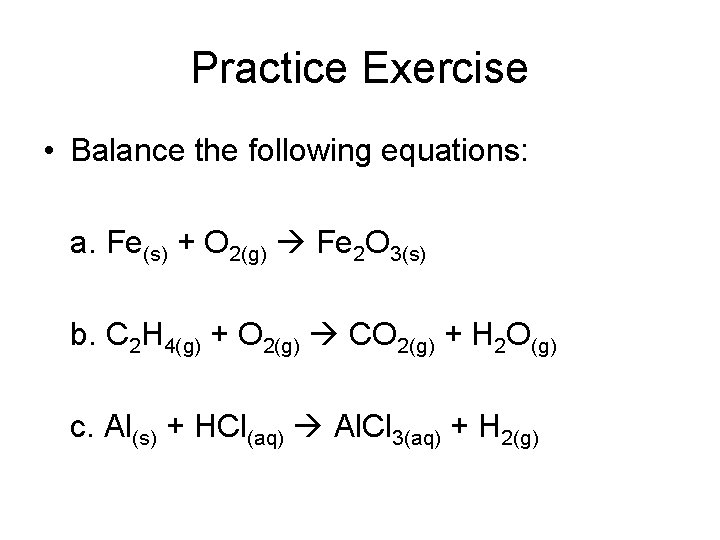Practice Exercise • Balance the following equations: a. Fe(s) + O 2(g) Fe 2 O 3(s) b. C 2 H 4(g) + O 2(g) CO 2(g) + H 2 O(g) c. Al(s) + HCl(aq) Al. Cl 3(aq) + H 2(g)Section 3. 2 – Some Simple Patterns of Chemical Reactivity • In synthesis/combination reactions two or more substances react to form one product. • Generic Reaction: A + B AB • Real Reaction: 2 Mg + O 2 2 Mg. ODecomposition Reactions • In a decomposition reaction one substance undergoes a reaction to produce two or more products. • Generic Reaction: AB A + B • Real Reaction: Ca. CO 3 Ca. O + CO 2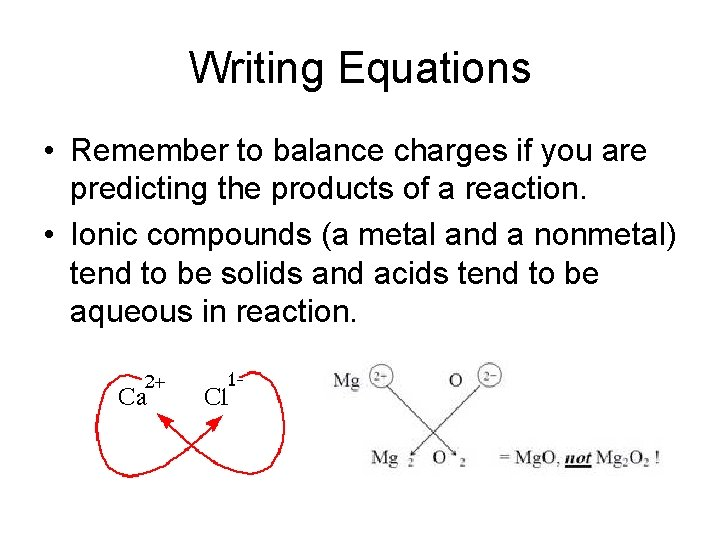Writing Equations • Remember to balance charges if you are predicting the products of a reaction. • Ionic compounds (a metal and a nonmetal) tend to be solids and acids tend to be aqueous in reaction.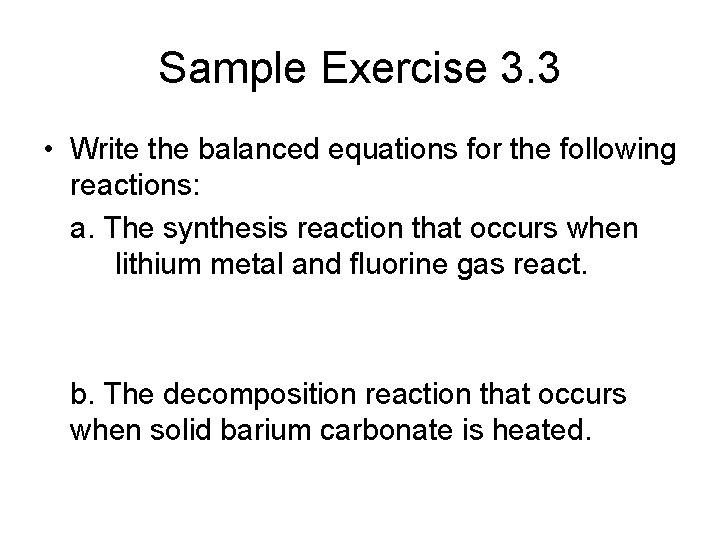Sample Exercise 3. 3 • Write the balanced equations for the following reactions: a. The synthesis reaction that occurs when lithium metal and fluorine gas react. b. The decomposition reaction that occurs when solid barium carbonate is heated.Hint for Predicting Products • Like in the previous exercise, carbonate ions tend to produce CO 2 when they break down.Practice Exercise • Write the balance equation for the following reactions: a. Solid mercury (II) sulfide decomposes into its elements when heated. b. The surface of aluminum metal undergoes a combination reaction with oxygen in the air.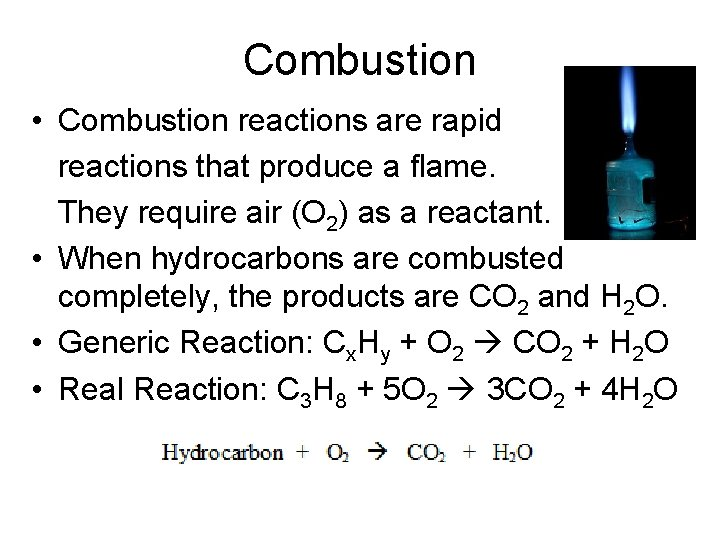Combustion • Combustion reactions are rapid reactions that produce a flame. They require air (O 2) as a reactant. • When hydrocarbons are combusted completely, the products are CO 2 and H 2 O. • Generic Reaction: Cx. Hy + O 2 CO 2 + H 2 O • Real Reaction: C 3 H 8 + 5 O 2 3 CO 2 + 4 H 2 O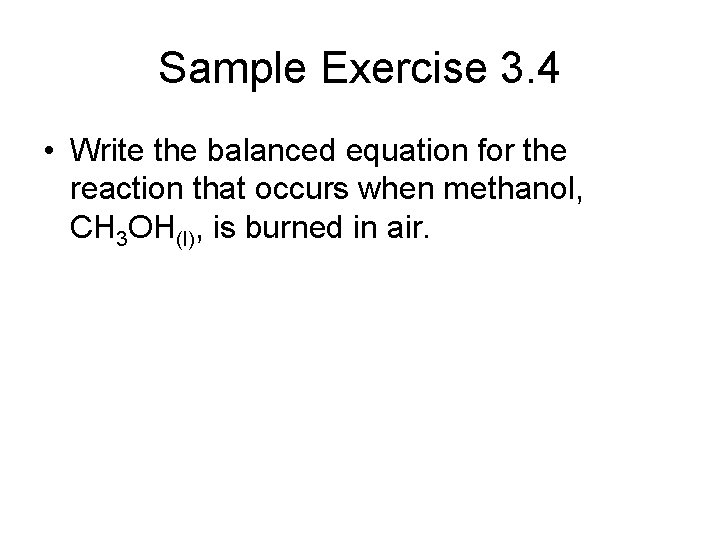Sample Exercise 3. 4 • Write the balanced equation for the reaction that occurs when methanol, CH 3 OH(l), is burned in air.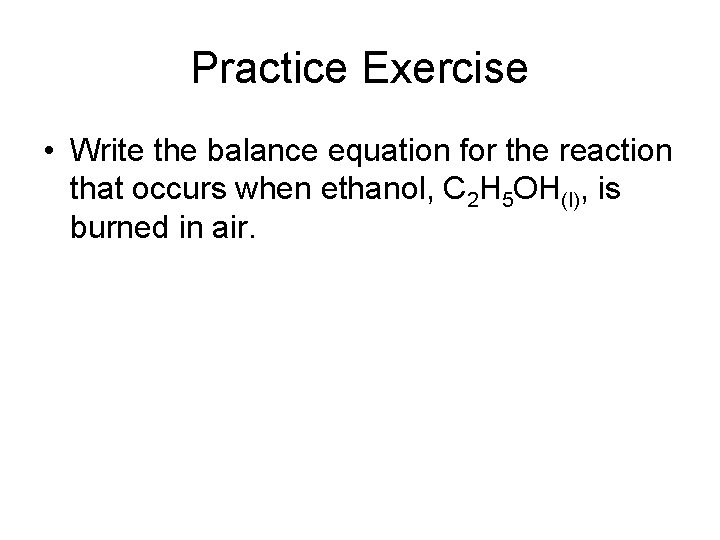Practice Exercise • Write the balance equation for the reaction that occurs when ethanol, C 2 H 5 OH(l), is burned in air.Formula Weights • The formula weight is a substance is the sum of the atomic weights of each atom in its chemical formula. • Ex: H 2 SO 4 = 2(1 amu) + 32 amu + 4(16 amu) = 98 amu § If the chemical formula is that of the molecule, then the formula weight is also called the molecular weight.Sample Exercise 3. 5 • Calculate the formula weight of a. sucrose (C 12 H 22 O 11) b. calcium nitrate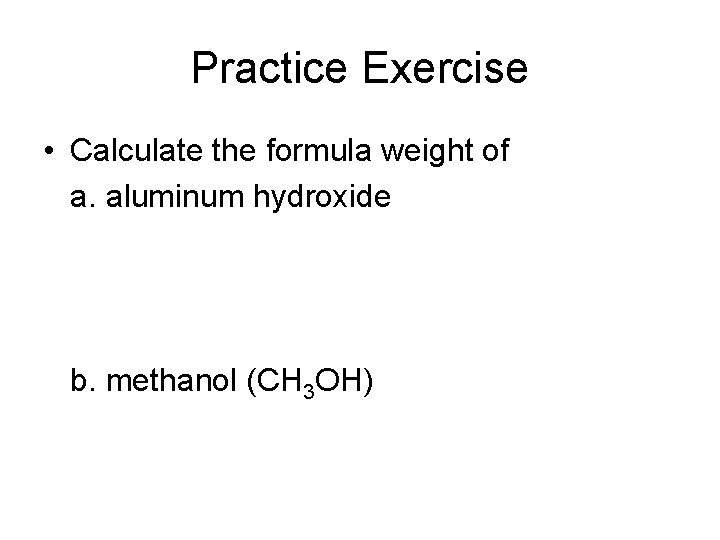Practice Exercise • Calculate the formula weight of a. aluminum hydroxide b. methanol (CH 3 OH)Percent Composition • Percent Composition is the percentage by mass contributed by each element in a substance. Percent Composition = mass of element x 100 mass of compound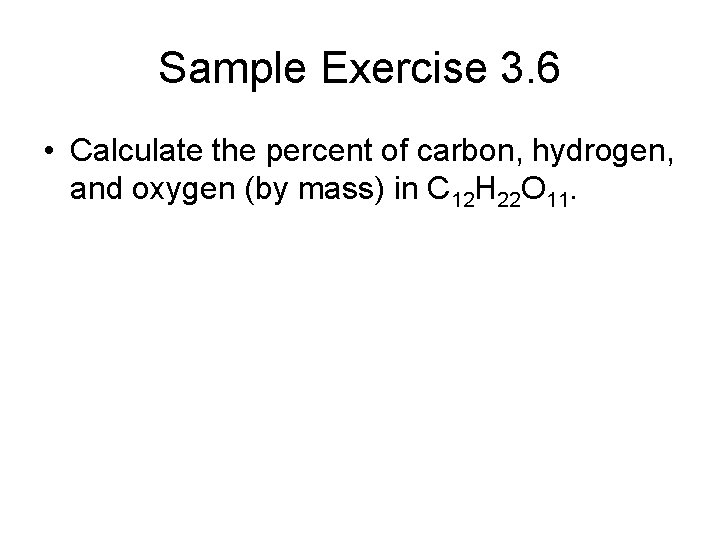Sample Exercise 3. 6 • Calculate the percent of carbon, hydrogen, and oxygen (by mass) in C 12 H 22 O 11.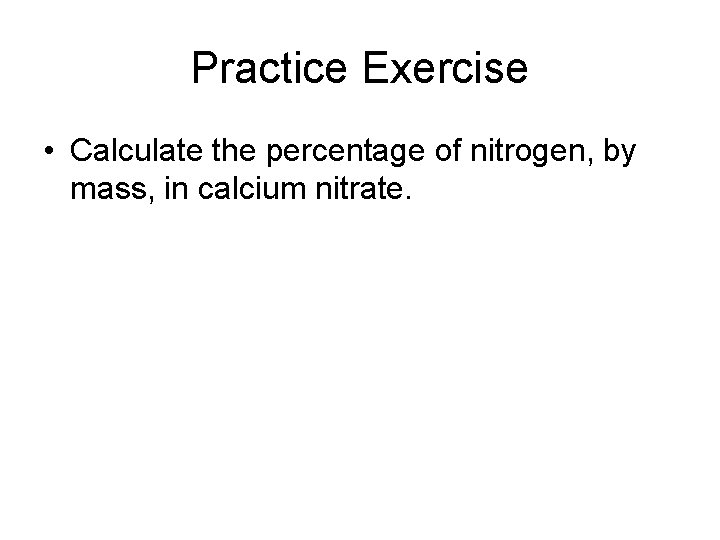Practice Exercise • Calculate the percentage of nitrogen, by mass, in calcium nitrate.Section 3. 4 – Avogadro’s Number and the Mole • In Chemistry the unit for dealing with the number of atoms, ions, or molecules in a common-sized sample is the mole, abbreviated mol. • 1 mol = 6. 02 x 1023 representative particlesSample Exercise 3. 7 • Arrange the following samples in order of increasing numbers of carbon atoms: 12 g C 12, 1 mol C 2 H 2, 9 x 1023 molecules of CO 2.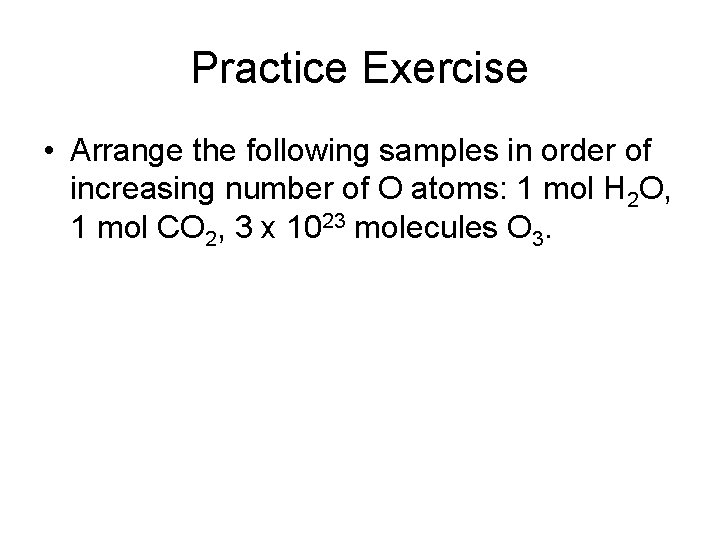Practice Exercise • Arrange the following samples in order of increasing number of O atoms: 1 mol H 2 O, 1 mol CO 2, 3 x 1023 molecules O 3.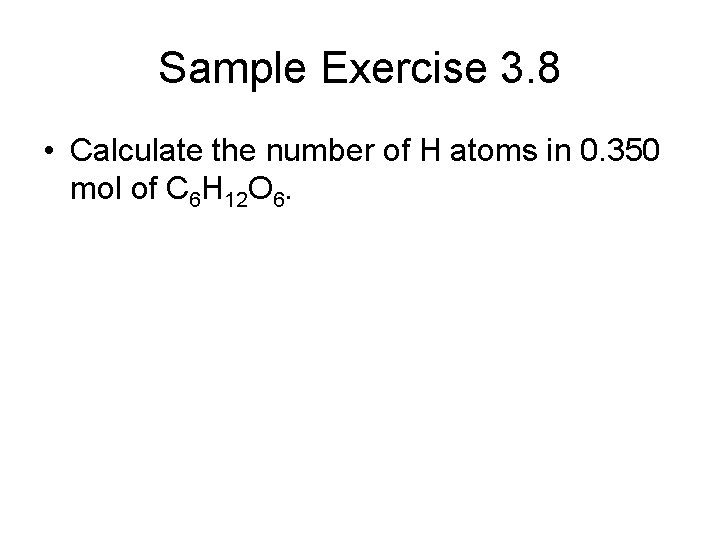Sample Exercise 3. 8 • Calculate the number of H atoms in 0. 350 mol of C 6 H 12 O 6.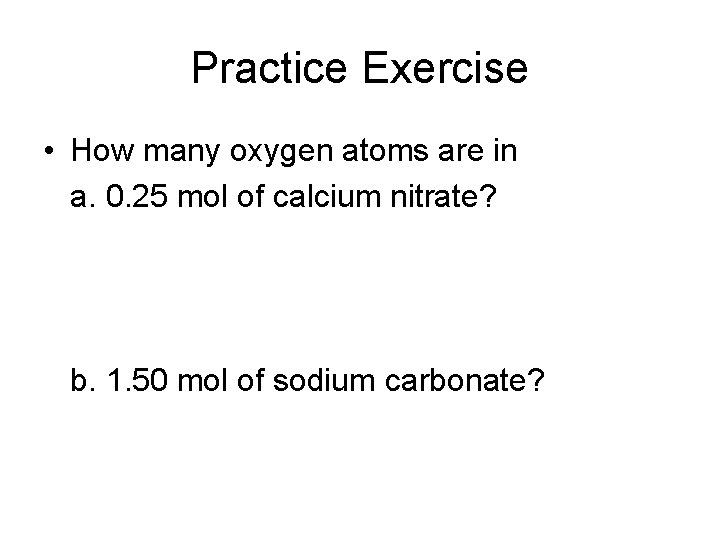Practice Exercise • How many oxygen atoms are in a. 0. 25 mol of calcium nitrate? b. 1. 50 mol of sodium carbonate?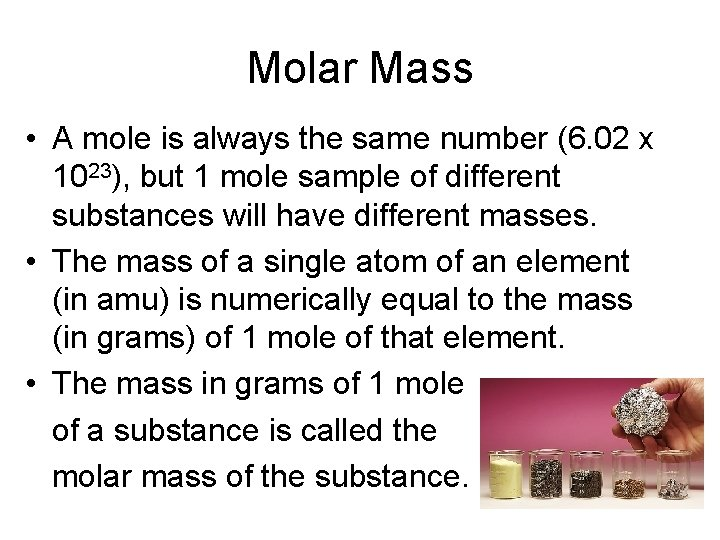Molar Mass • A mole is always the same number (6. 02 x 1023), but 1 mole sample of different substances will have different masses. • The mass of a single atom of an element (in amu) is numerically equal to the mass (in grams) of 1 mole of that element. • The mass in grams of 1 mole of a substance is called the molar mass of the substance.Sample Exercise 3. 9 • What is the mass in grams of 1. 000 mol of glucose, C 6 H 12 O 6?Practice Exercise • Calculate the molar mass of calcium nitrate.Sample Exercise 3. 10 • Calculate the number of moles of glucose in 5. 380 g of glucose.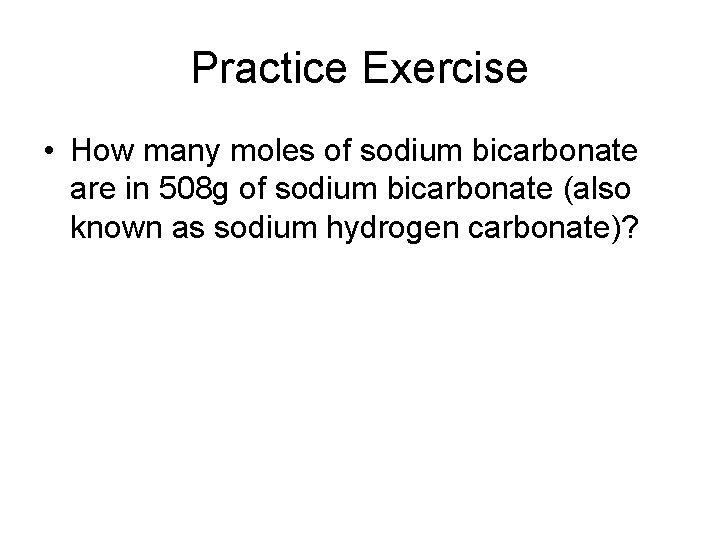Practice Exercise • How many moles of sodium bicarbonate are in 508 g of sodium bicarbonate (also known as sodium hydrogen carbonate)?Sample Exercise 3. 11 • Calculate the mass, in grams. Of 0. 433 mol of calcium nitrate.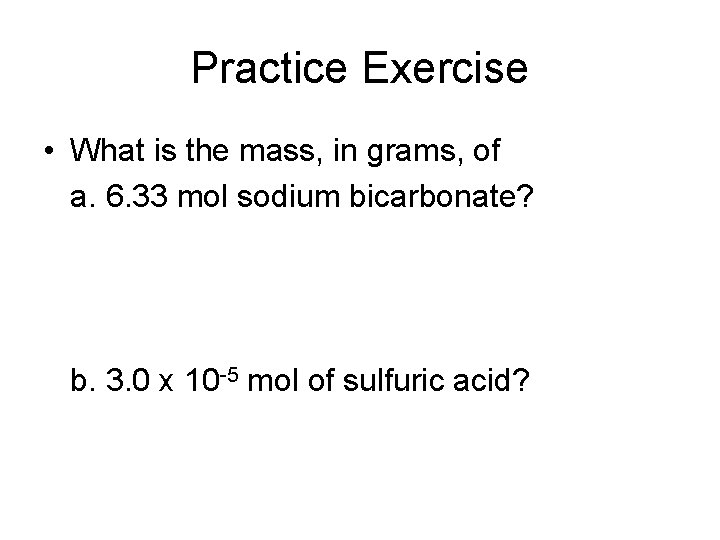Practice Exercise • What is the mass, in grams, of a. 6. 33 mol sodium bicarbonate? b. 3. 0 x 10 -5 mol of sulfuric acid?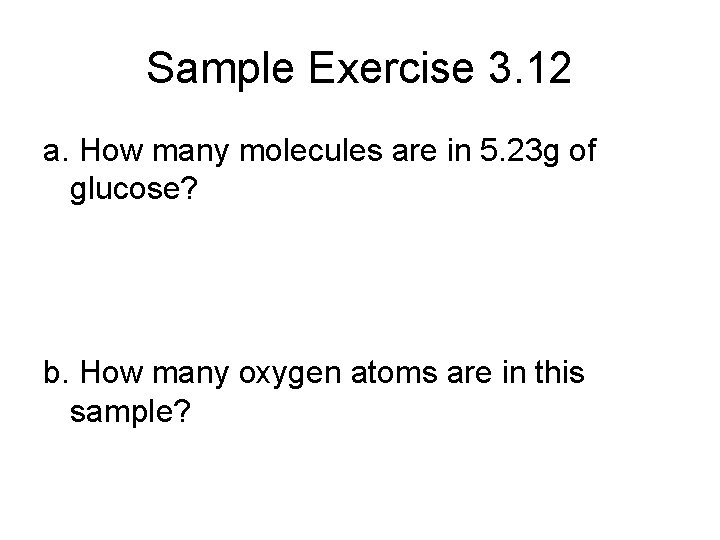Sample Exercise 3. 12 a. How many molecules are in 5. 23 g of glucose? b. How many oxygen atoms are in this sample?Practice Exercise a. How many molecules are in 4. 20 g of nitric acid? b. How many O atoms are in this sample?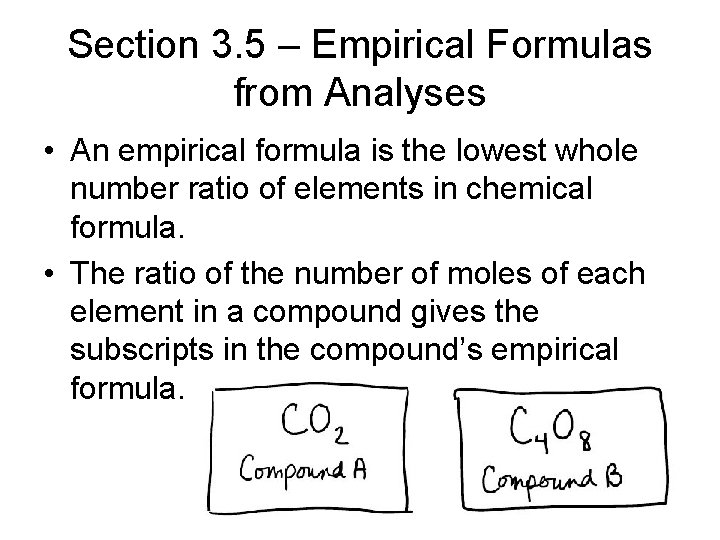Section 3. 5 – Empirical Formulas from Analyses • An empirical formula is the lowest whole number ratio of elements in chemical formula. • The ratio of the number of moles of each element in a compound gives the subscripts in the compound’s empirical formula.Empirical Formula 1. When given percentages, assume 100 g so that the percentages can be grams. 2. Divide the mass of each element by its molar mass. 3. Divide all answers by the lowest number to get subscripts. 4. If you get a. 5, then multiply all numbers by 2. If you get a. 33 or. 66, then multiply all numbers by 3.Sample Exercise 3. 13 § Ascorbic acid (vitamin C) contains 40. 92% C, 4. 58% H, and 54. 5% O by mass. What is the empirical formula of ascorbic acid?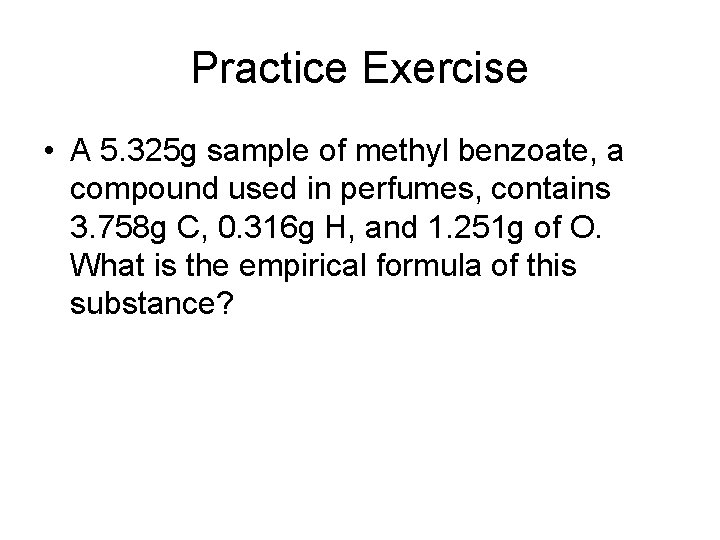Practice Exercise • A 5. 325 g sample of methyl benzoate, a compound used in perfumes, contains 3. 758 g C, 0. 316 g H, and 1. 251 g of O. What is the empirical formula of this substance?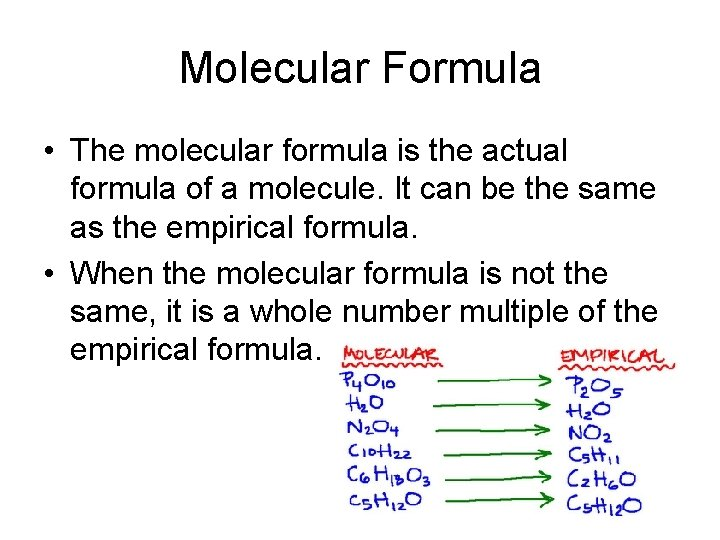Molecular Formula • The molecular formula is the actual formula of a molecule. It can be the same as the empirical formula. • When the molecular formula is not the same, it is a whole number multiple of the empirical formula.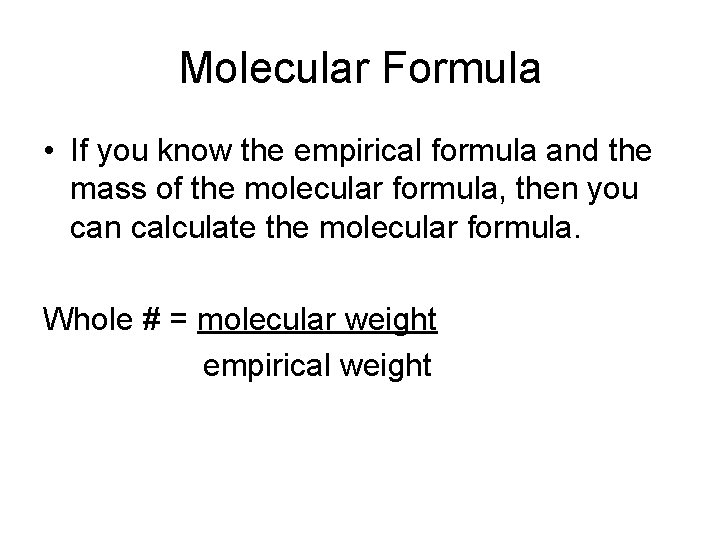Molecular Formula • If you know the empirical formula and the mass of the molecular formula, then you can calculate the molecular formula. Whole # = molecular weight empirical weightSample Exercise 3. 14 • Mesitylene, a hydrocarbon in crude oil, has an empirical formula of C 3 H 4. The molecular weight of the substance is 121 amu. What is the molecular formula of mesitylene?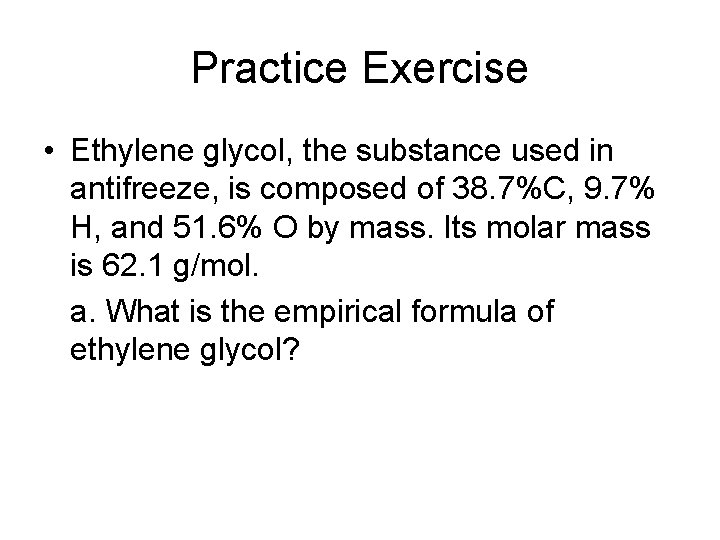Practice Exercise • Ethylene glycol, the substance used in antifreeze, is composed of 38. 7%C, 9. 7% H, and 51. 6% O by mass. Its molar mass is 62. 1 g/mol. a. What is the empirical formula of ethylene glycol?Practice Exercise b. What is the molecular formula for the previous question?Combustion Analysis • For combustion reactions, we can calculate the number of moles (subscripts) of C and H from the amounts of CO 2 and H 2 O. • If any other elements are present, then we can subtract that mass to get the mass of only C and H.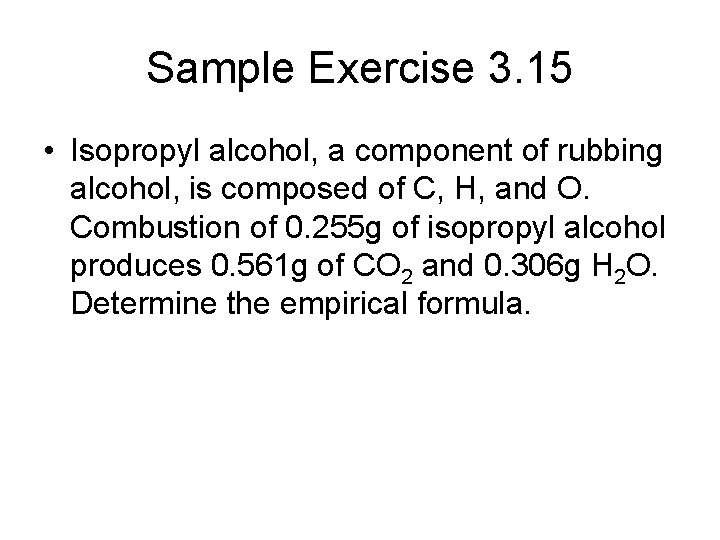Sample Exercise 3. 15 • Isopropyl alcohol, a component of rubbing alcohol, is composed of C, H, and O. Combustion of 0. 255 g of isopropyl alcohol produces 0. 561 g of CO 2 and 0. 306 g H 2 O. Determine the empirical formula.Practice Exercise a. Caproic acid, the smell in dirty socks, is composed of C, H, and O atoms. Combustion of a 0. 225 g sample of this compound produces 0. 512 g CO 2, 0. 209 g H 2 O. What is the empirical formula of caproic acid?Practice Exercise b. Caproic acid has a molar mass of 116 g/mol. What is the molecular formula?Section 3. 6 – Quantitative Information from Balanced Equations • The coefficients in a balanced equation indicate both the relative numbers of moles in the reaction. • A mole ratio is the ratio of the coefficients of 2 substances in a chemical equation. • You must use the mole ratio to convert from one substance to another.Sample Exercise 3. 16 • How many grams of water are produced in the oxidation of 1. 00 g of glucose? C 6 H 12 O 6(s) + 6 O 2(g) 6 CO 2(g) + 6 O 2(g)Practice Exercise • How many grams of O 2 can be prepared from 4. 50 g KCl. O 3? 2 KCl. O 3(s) 2 KCl(s) + 3 O 2(g)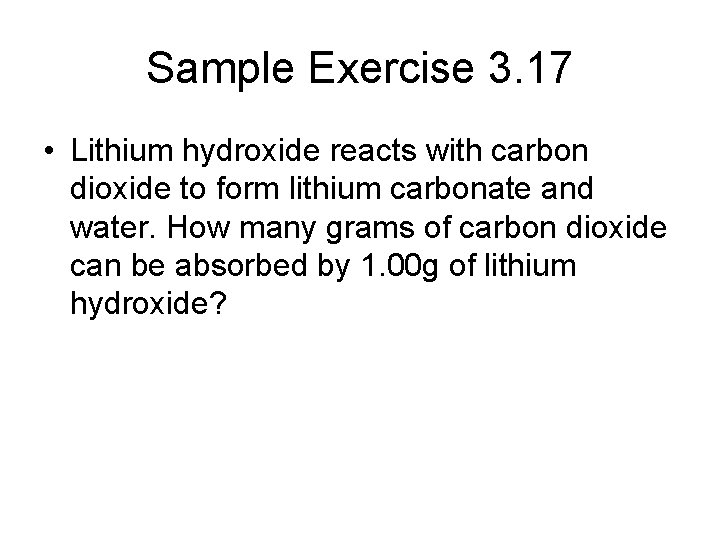Sample Exercise 3. 17 • Lithium hydroxide reacts with carbon dioxide to form lithium carbonate and water. How many grams of carbon dioxide can be absorbed by 1. 00 g of lithium hydroxide?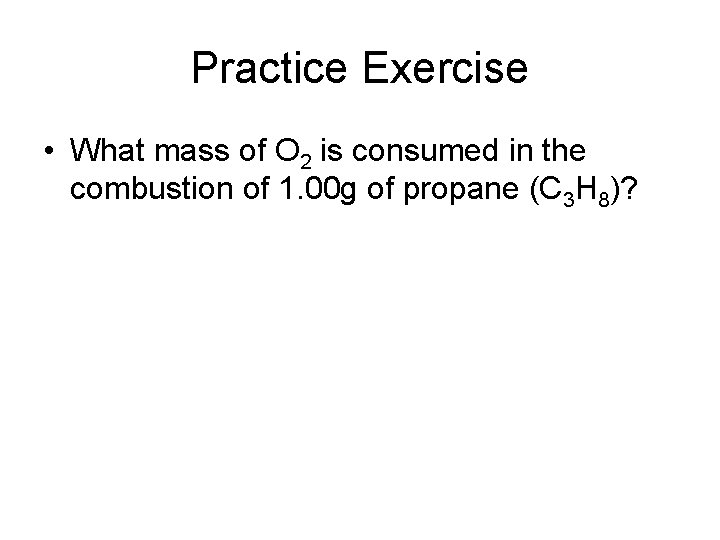Practice Exercise • What mass of O 2 is consumed in the combustion of 1. 00 g of propane (C 3 H 8)?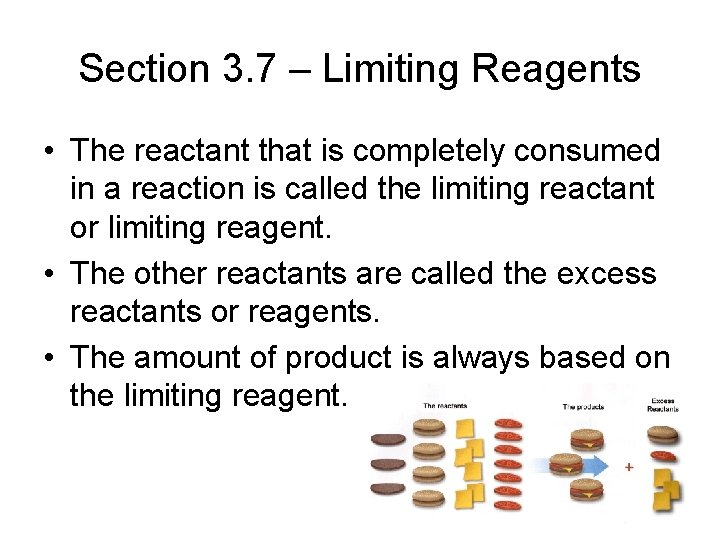Section 3. 7 – Limiting Reagents • The reactant that is completely consumed in a reaction is called the limiting reactant or limiting reagent. • The other reactants are called the excess reactants or reagents. • The amount of product is always based on the limiting reagent.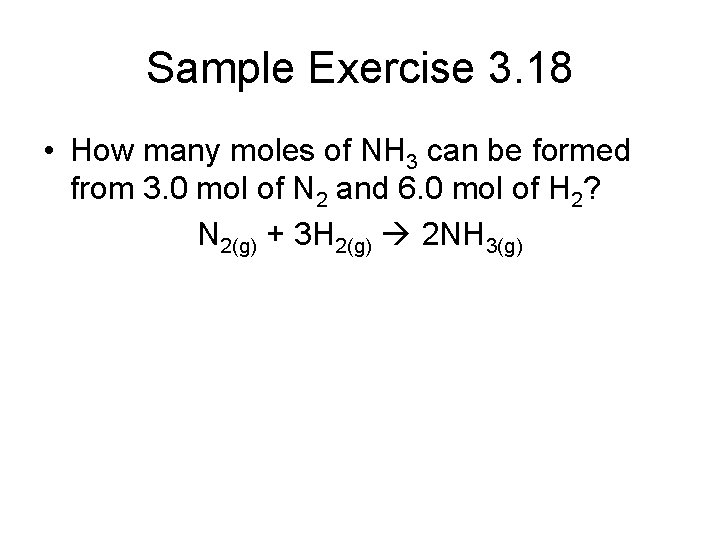Sample Exercise 3. 18 • How many moles of NH 3 can be formed from 3. 0 mol of N 2 and 6. 0 mol of H 2? N 2(g) + 3 H 2(g) 2 NH 3(g)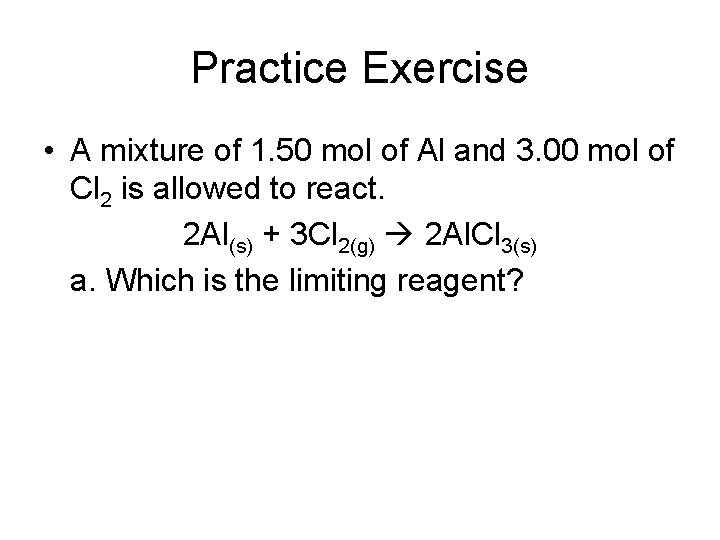Practice Exercise • A mixture of 1. 50 mol of Al and 3. 00 mol of Cl 2 is allowed to react. 2 Al(s) + 3 Cl 2(g) 2 Al. Cl 3(s) a. Which is the limiting reagent?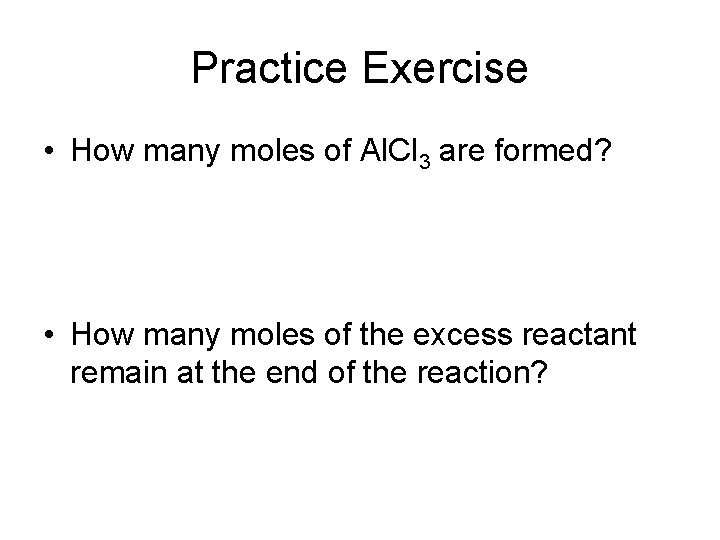Practice Exercise • How many moles of Al. Cl 3 are formed? • How many moles of the excess reactant remain at the end of the reaction?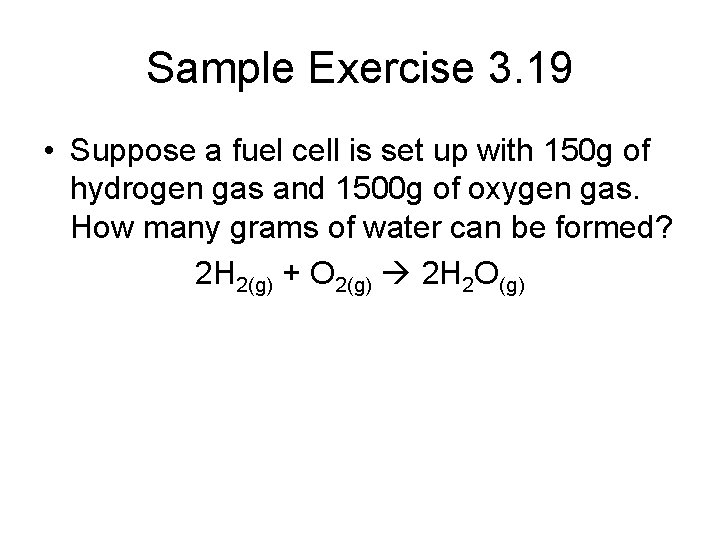Sample Exercise 3. 19 • Suppose a fuel cell is set up with 150 g of hydrogen gas and 1500 g of oxygen gas. How many grams of water can be formed? 2 H 2(g) + O 2(g) 2 H 2 O(g)Practice Exercise • A strip of zinc metal with a mass of 2. 00 g is placed in an aqueous solution containing 2. 50 g of silver nitrate, causing the following reaction to occur: Zn(s) + 2 Ag. NO 3(aq) 2 Ag(s) + Zn(NO 3)2(aq) a. Which reactant is limiting?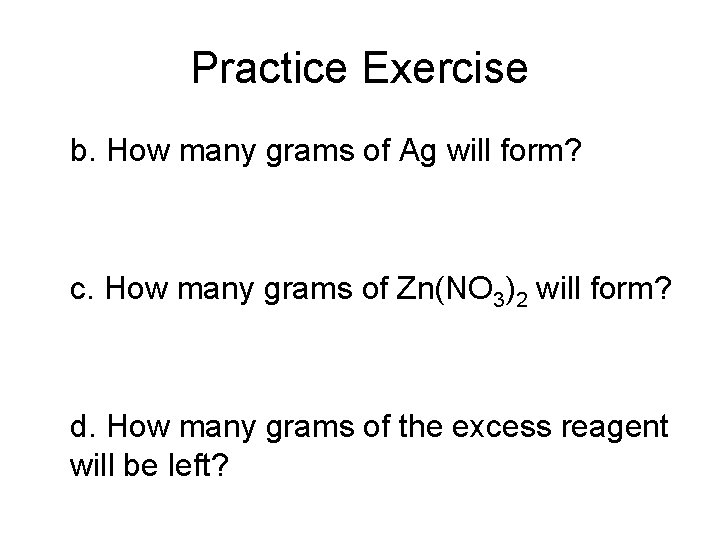Practice Exercise b. How many grams of Ag will form? c. How many grams of Zn(NO 3)2 will form? d. How many grams of the excess reagent will be left?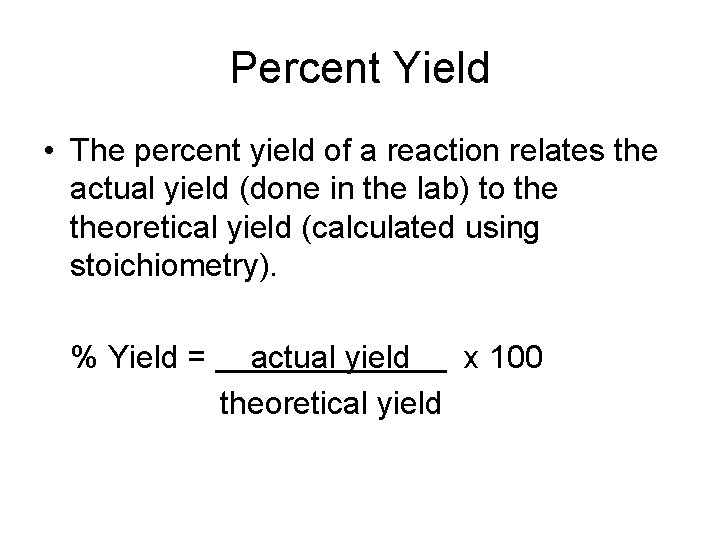Percent Yield • The percent yield of a reaction relates the actual yield (done in the lab) to theoretical yield (calculated using stoichiometry). % Yield = actual yield x 100 theoretical yield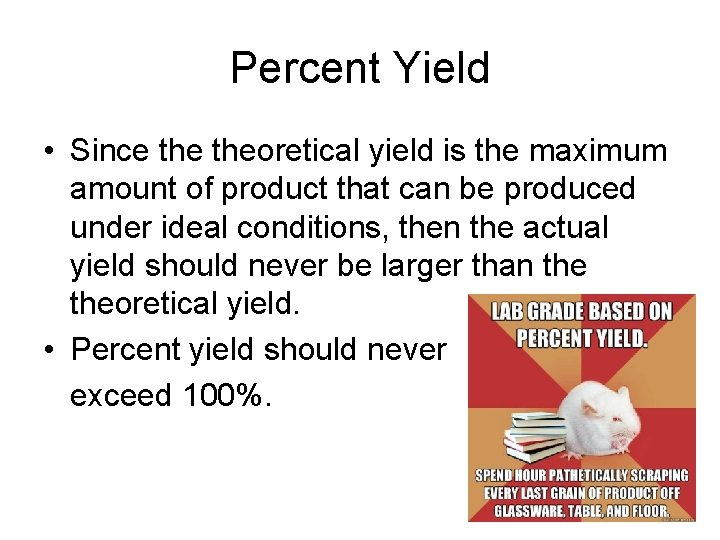Percent Yield • Since theoretical yield is the maximum amount of product that can be produced under ideal conditions, then the actual yield should never be larger than theoretical yield. • Percent yield should never exceed 100%.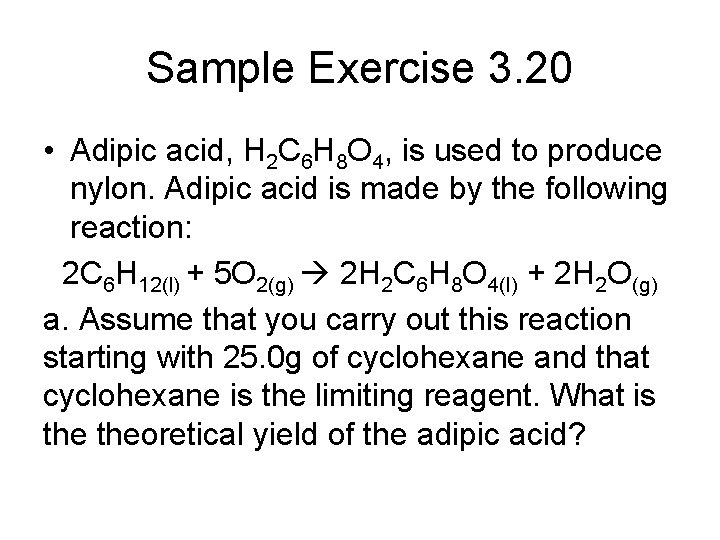Sample Exercise 3. 20 • Adipic acid, H 2 C 6 H 8 O 4, is used to produce nylon. Adipic acid is made by the following reaction: 2 C 6 H 12(l) + 5 O 2(g) 2 H 2 C 6 H 8 O 4(l) + 2 H 2 O(g) a. Assume that you carry out this reaction starting with 25. 0 g of cyclohexane and that cyclohexane is the limiting reagent. What is theoretical yield of the adipic acid?Sample Exercise 3. 20 b. If you obtain 33. 5 g of adipic acid from your reaction, what is the percent yield of adipic acid?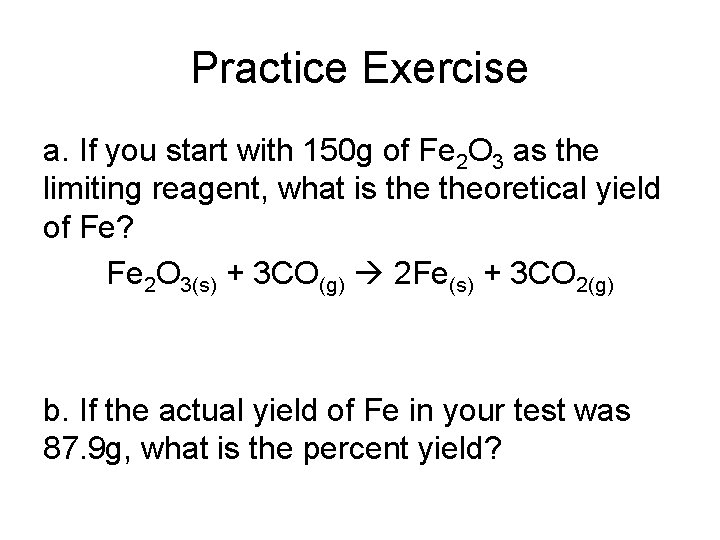Practice Exercise a. If you start with 150 g of Fe 2 O 3 as the limiting reagent, what is theoretical yield of Fe? Fe 2 O 3(s) + 3 CO(g) 2 Fe(s) + 3 CO 2(g) b. If the actual yield of Fe in your test was 87. 9 g, what is the percent yield?